# CutnellJohnson Physics 7 th edition Classroom Response System

• Slides: 31Cutnell/Johnson Physics 7 th edition Classroom Response System Questions Chapter 5 Dynamics of Uniform Circular Motion Reading Quiz Questions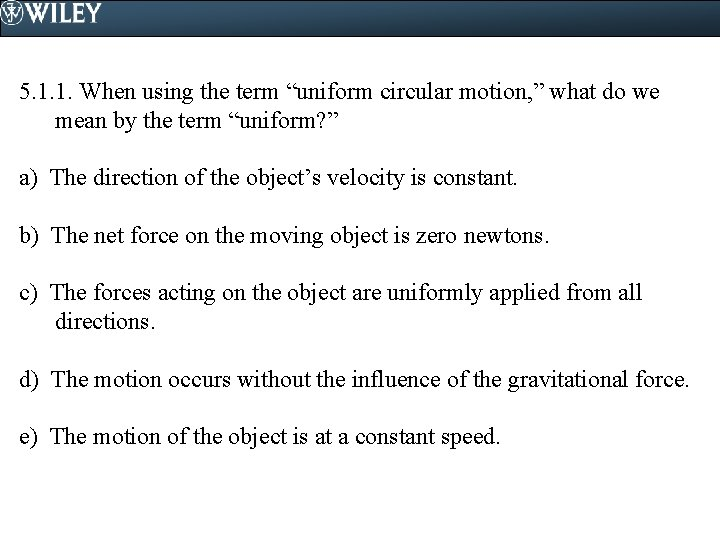5. 1. 1. When using the term “uniform circular motion, ” what do we mean by the term “uniform? ” a) The direction of the object’s velocity is constant. b) The net force on the moving object is zero newtons. c) The forces acting on the object are uniformly applied from all directions. d) The motion occurs without the influence of the gravitational force. e) The motion of the object is at a constant speed.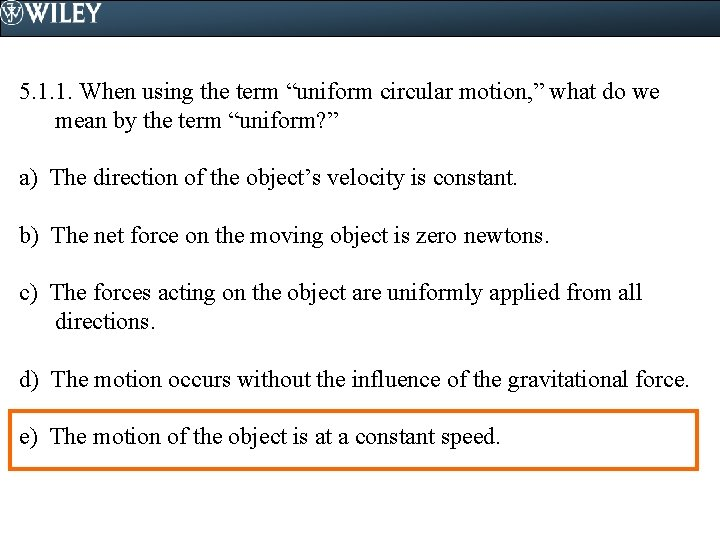5. 1. 1. When using the term “uniform circular motion, ” what do we mean by the term “uniform? ” a) The direction of the object’s velocity is constant. b) The net force on the moving object is zero newtons. c) The forces acting on the object are uniformly applied from all directions. d) The motion occurs without the influence of the gravitational force. e) The motion of the object is at a constant speed.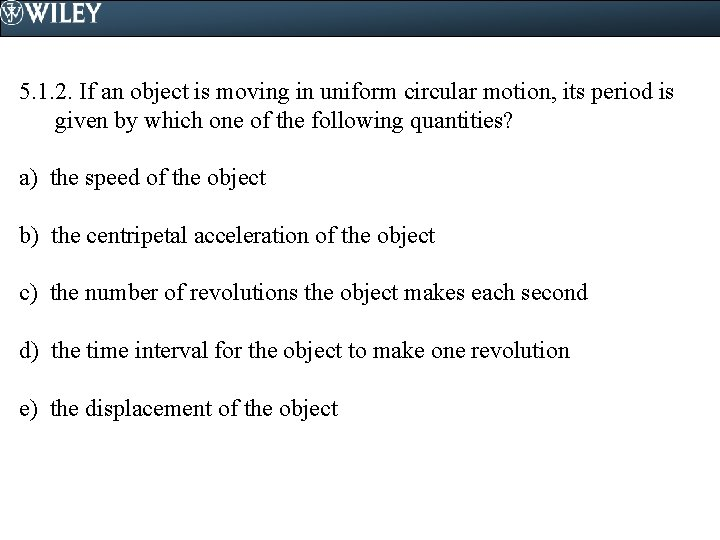5. 1. 2. If an object is moving in uniform circular motion, its period is given by which one of the following quantities? a) the speed of the object b) the centripetal acceleration of the object c) the number of revolutions the object makes each second d) the time interval for the object to make one revolution e) the displacement of the object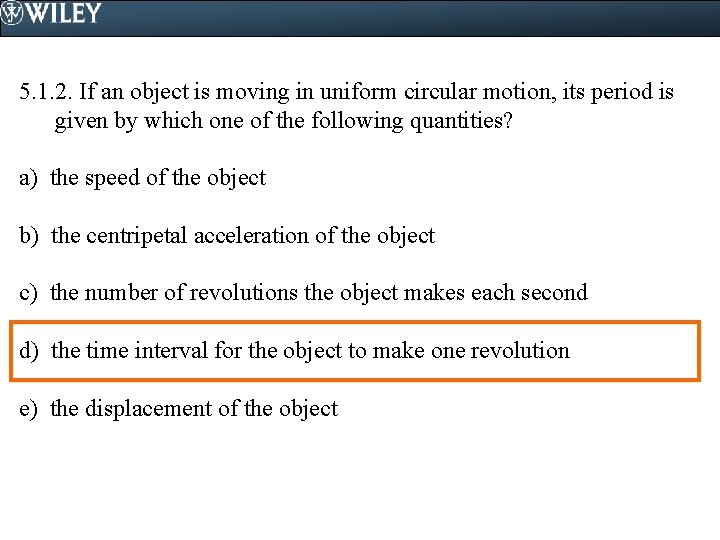5. 1. 2. If an object is moving in uniform circular motion, its period is given by which one of the following quantities? a) the speed of the object b) the centripetal acceleration of the object c) the number of revolutions the object makes each second d) the time interval for the object to make one revolution e) the displacement of the object5. 2. 1. A bicycle racer is traveling at constant speed v around a circular track. The centripetal acceleration of the bicycle is ac. What happens to the centripetal acceleration of the bicycle if the speed is doubled to 2 v? a) The centripetal acceleration increases to 4 ac. b) The centripetal acceleration decreases to 0. 25 ac. c) The centripetal acceleration increases to 2 ac. d) The centripetal acceleration decreases to 0. 5 ac. e) The centripetal acceleration does not change.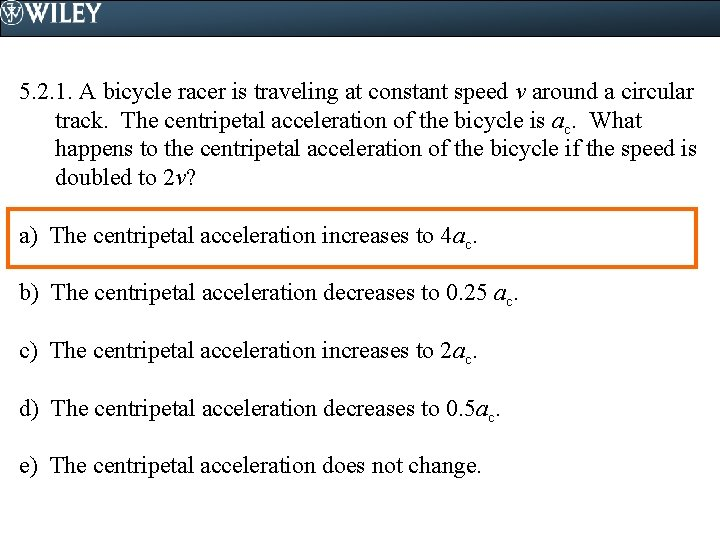5. 2. 1. A bicycle racer is traveling at constant speed v around a circular track. The centripetal acceleration of the bicycle is ac. What happens to the centripetal acceleration of the bicycle if the speed is doubled to 2 v? a) The centripetal acceleration increases to 4 ac. b) The centripetal acceleration decreases to 0. 25 ac. c) The centripetal acceleration increases to 2 ac. d) The centripetal acceleration decreases to 0. 5 ac. e) The centripetal acceleration does not change.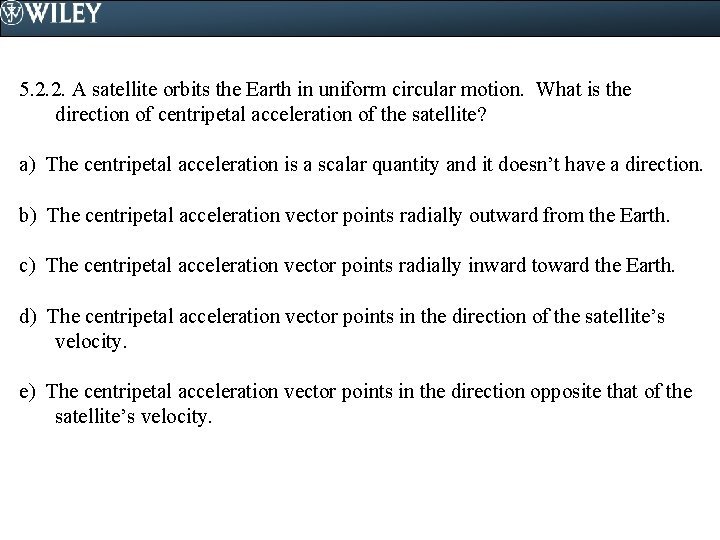5. 2. 2. A satellite orbits the Earth in uniform circular motion. What is the direction of centripetal acceleration of the satellite? a) The centripetal acceleration is a scalar quantity and it doesn’t have a direction. b) The centripetal acceleration vector points radially outward from the Earth. c) The centripetal acceleration vector points radially inward toward the Earth. d) The centripetal acceleration vector points in the direction of the satellite’s velocity. e) The centripetal acceleration vector points in the direction opposite that of the satellite’s velocity.5. 2. 2. A satellite orbits the Earth in uniform circular motion. What is the direction of centripetal acceleration of the satellite? a) The centripetal acceleration is a scalar quantity and it doesn’t have a direction. b) The centripetal acceleration vector points radially outward from the Earth. c) The centripetal acceleration vector points radially inward toward the Earth. d) The centripetal acceleration vector points in the direction of the satellite’s velocity. e) The centripetal acceleration vector points in the direction opposite that of the satellite’s velocity.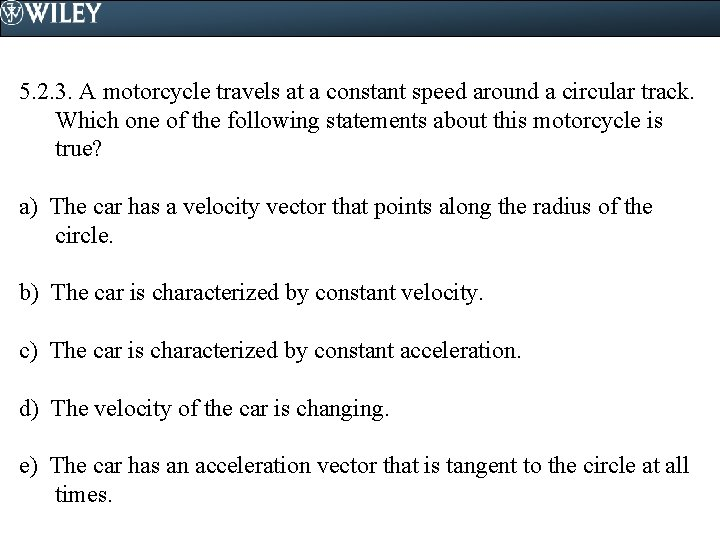5. 2. 3. A motorcycle travels at a constant speed around a circular track. Which one of the following statements about this motorcycle is true? a) The car has a velocity vector that points along the radius of the circle. b) The car is characterized by constant velocity. c) The car is characterized by constant acceleration. d) The velocity of the car is changing. e) The car has an acceleration vector that is tangent to the circle at all times.5. 2. 3. A motorcycle travels at a constant speed around a circular track. Which one of the following statements about this motorcycle is true? a) The car has a velocity vector that points along the radius of the circle. b) The car is characterized by constant velocity. c) The car is characterized by constant acceleration. d) The velocity of the car is changing. e) The car has an acceleration vector that is tangent to the circle at all times.5. 2. 4. A truck is traveling with a constant speed of 15 m/s. When the truck follows a curve in the road, its centripetal acceleration is 4. 0 m/s 2. What is the radius of the curve? a) 3. 8 m b) 14 m c) 56 m d) 120 m e) 210 m5. 2. 4. A truck is traveling with a constant speed of 15 m/s. When the truck follows a curve in the road, its centripetal acceleration is 4. 0 m/s 2. What is the radius of the curve? a) 3. 8 m b) 14 m c) 56 m d) 120 m e) 210 m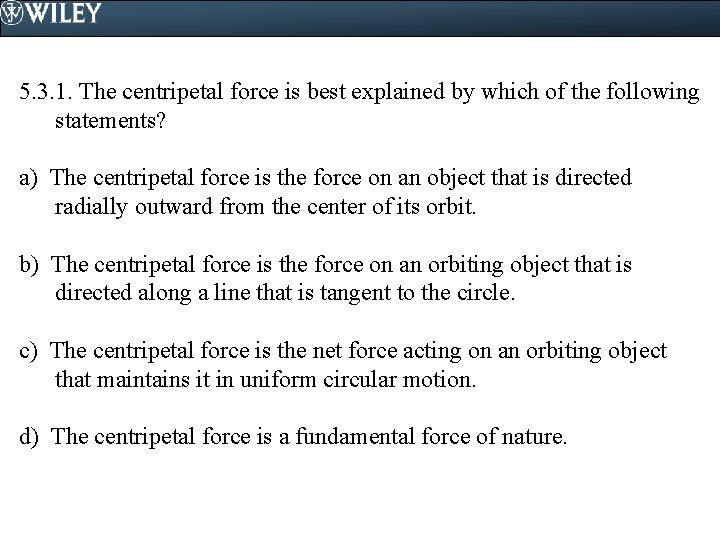5. 3. 1. The centripetal force is best explained by which of the following statements? a) The centripetal force is the force on an object that is directed radially outward from the center of its orbit. b) The centripetal force is the force on an orbiting object that is directed along a line that is tangent to the circle. c) The centripetal force is the net force acting on an orbiting object that maintains it in uniform circular motion. d) The centripetal force is a fundamental force of nature.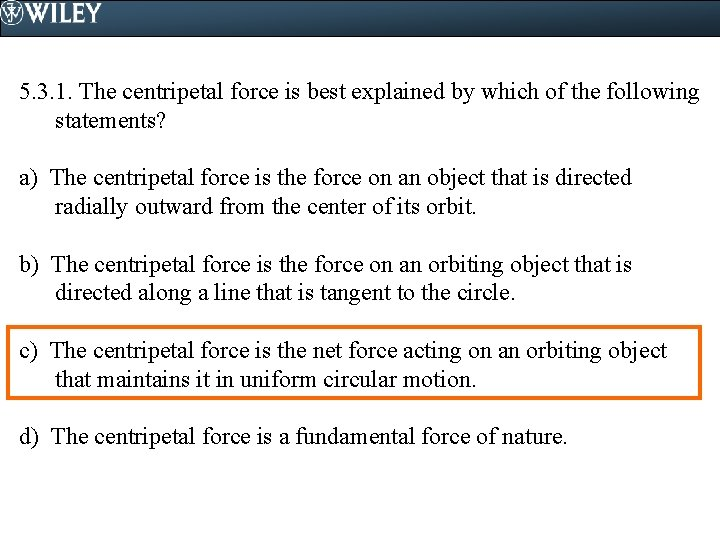5. 3. 1. The centripetal force is best explained by which of the following statements? a) The centripetal force is the force on an object that is directed radially outward from the center of its orbit. b) The centripetal force is the force on an orbiting object that is directed along a line that is tangent to the circle. c) The centripetal force is the net force acting on an orbiting object that maintains it in uniform circular motion. d) The centripetal force is a fundamental force of nature.5. 4. 1. Which one of the following forces holds a car on a frictionless banked curve? a) the vertical component of the normal force b) the vertical component of the car's weight c) the horizontal component of the normal force d) the horizontal component of the car's weight e) the reaction force to the car's weight5. 4. 1. Which one of the following forces holds a car on a frictionless banked curve? a) the vertical component of the normal force b) the vertical component of the car's weight c) the horizontal component of the normal force d) the horizontal component of the car's weight e) the reaction force to the car's weight5. 4. 2. The maximum speed at which a car can safely negotiate a frictionless banked curve depends on all of the following except a) the acceleration due to gravity. b) the radius of the curve. c) the angle of banking. d) the diameter of the curve. e) the mass of the car.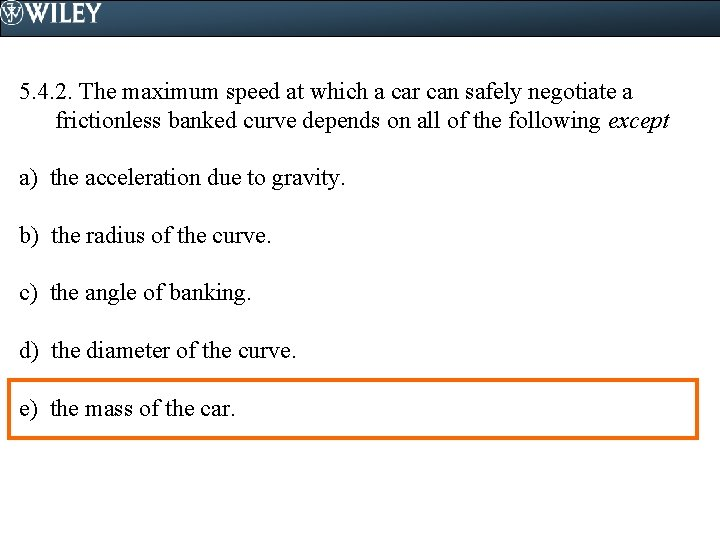5. 4. 2. The maximum speed at which a car can safely negotiate a frictionless banked curve depends on all of the following except a) the acceleration due to gravity. b) the radius of the curve. c) the angle of banking. d) the diameter of the curve. e) the mass of the car.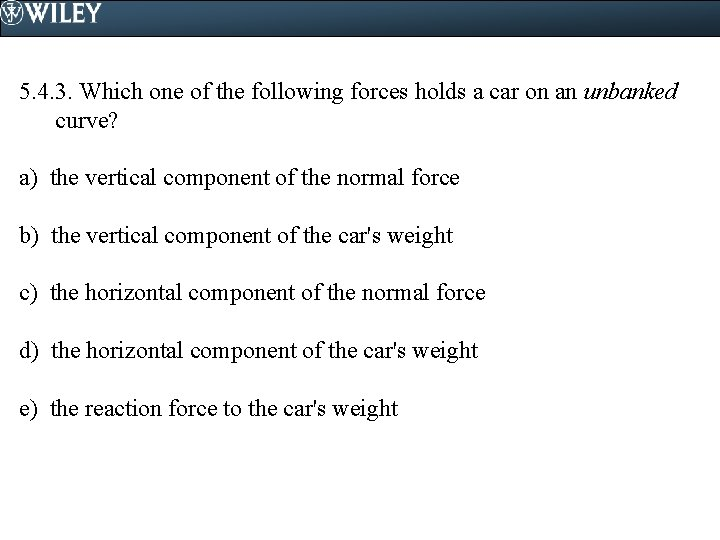5. 4. 3. Which one of the following forces holds a car on an unbanked curve? a) the vertical component of the normal force b) the vertical component of the car's weight c) the horizontal component of the normal force d) the horizontal component of the car's weight e) the reaction force to the car's weight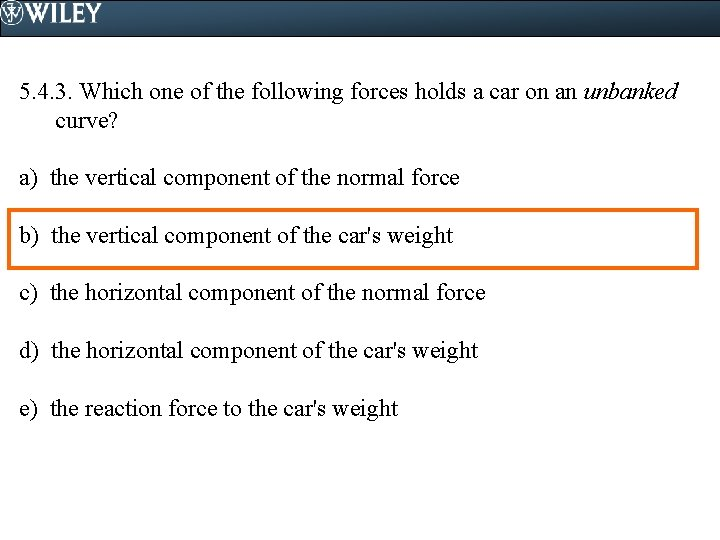5. 4. 3. Which one of the following forces holds a car on an unbanked curve? a) the vertical component of the normal force b) the vertical component of the car's weight c) the horizontal component of the normal force d) the horizontal component of the car's weight e) the reaction force to the car's weight5. 5. 1. The period of a satellite orbiting Earth is not dependent on which one of the following quantities? a) the mass of the satellite b) the mass of the Earth c) the distance from the center of the Earth to the satellite d) G, the universal gravitational constant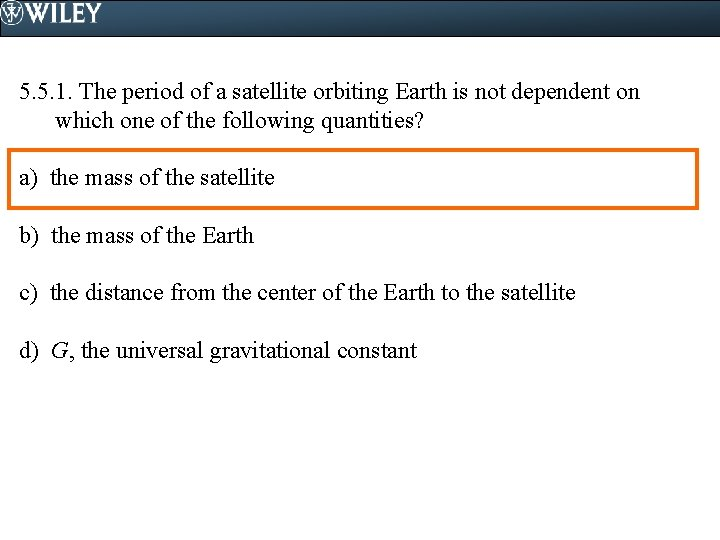5. 5. 1. The period of a satellite orbiting Earth is not dependent on which one of the following quantities? a) the mass of the satellite b) the mass of the Earth c) the distance from the center of the Earth to the satellite d) G, the universal gravitational constant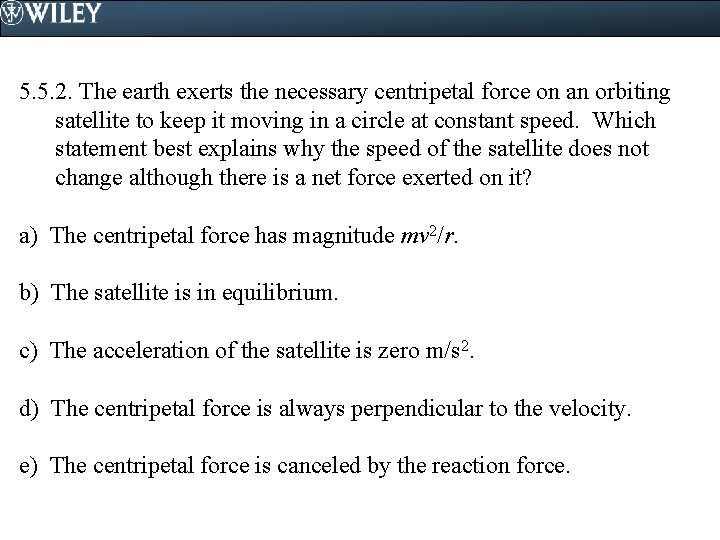5. 5. 2. The earth exerts the necessary centripetal force on an orbiting satellite to keep it moving in a circle at constant speed. Which statement best explains why the speed of the satellite does not change although there is a net force exerted on it? a) The centripetal force has magnitude mv 2/r. b) The satellite is in equilibrium. c) The acceleration of the satellite is zero m/s 2. d) The centripetal force is always perpendicular to the velocity. e) The centripetal force is canceled by the reaction force.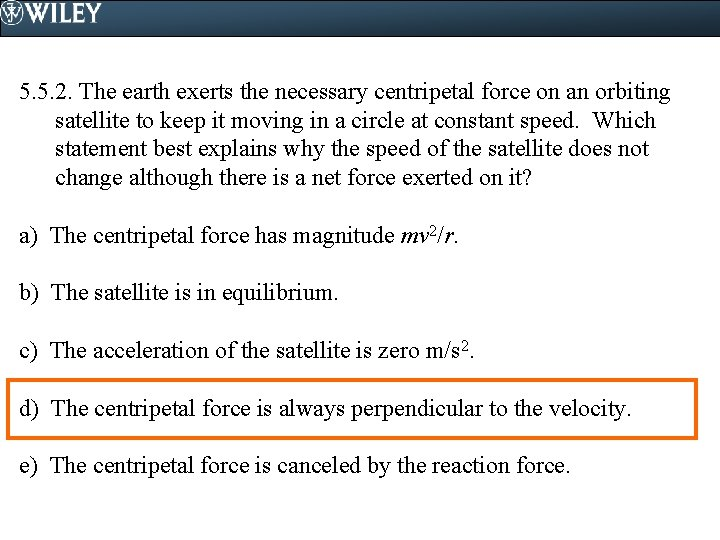5. 5. 2. The earth exerts the necessary centripetal force on an orbiting satellite to keep it moving in a circle at constant speed. Which statement best explains why the speed of the satellite does not change although there is a net force exerted on it? a) The centripetal force has magnitude mv 2/r. b) The satellite is in equilibrium. c) The acceleration of the satellite is zero m/s 2. d) The centripetal force is always perpendicular to the velocity. e) The centripetal force is canceled by the reaction force.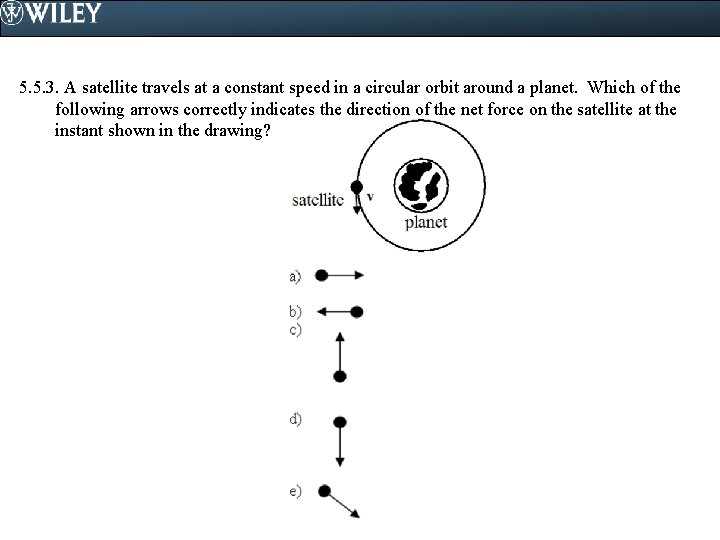5. 5. 3. A satellite travels at a constant speed in a circular orbit around a planet. Which of the following arrows correctly indicates the direction of the net force on the satellite at the instant shown in the drawing?5. 5. 3. A satellite travels at a constant speed in a circular orbit around a planet. Which of the following arrows correctly indicates the direction of the net force on the satellite at the instant shown in the drawing?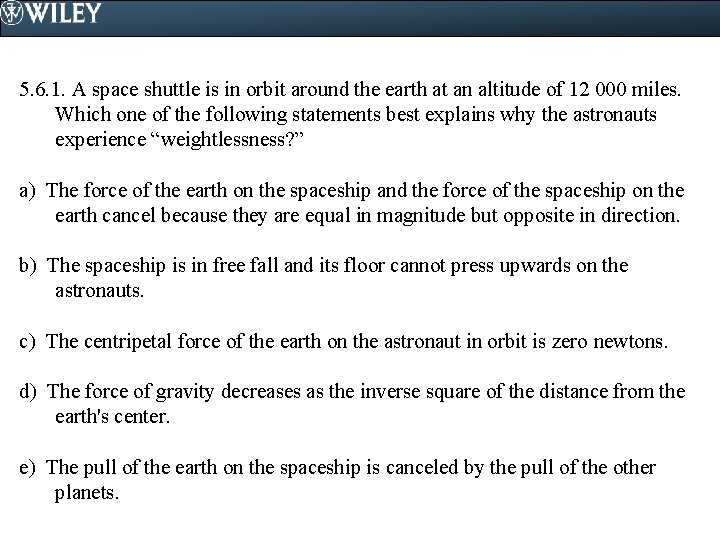5. 6. 1. A space shuttle is in orbit around the earth at an altitude of 12 000 miles. Which one of the following statements best explains why the astronauts experience “weightlessness? ” a) The force of the earth on the spaceship and the force of the spaceship on the earth cancel because they are equal in magnitude but opposite in direction. b) The spaceship is in free fall and its floor cannot press upwards on the astronauts. c) The centripetal force of the earth on the astronaut in orbit is zero newtons. d) The force of gravity decreases as the inverse square of the distance from the earth's center. e) The pull of the earth on the spaceship is canceled by the pull of the other planets.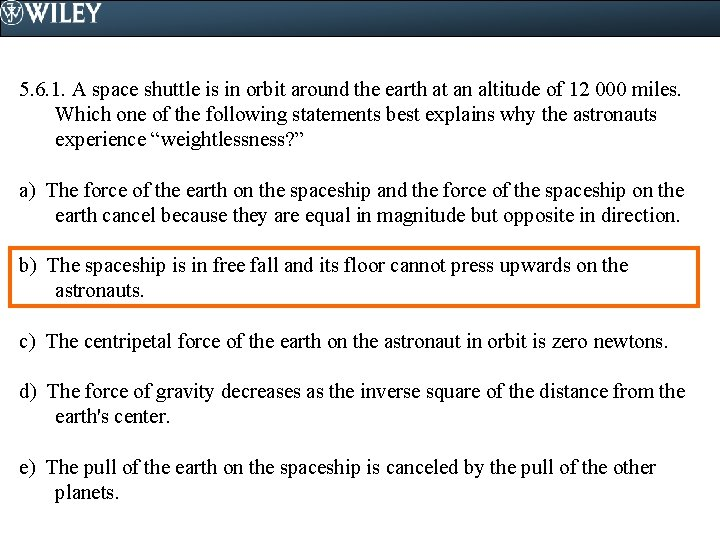5. 6. 1. A space shuttle is in orbit around the earth at an altitude of 12 000 miles. Which one of the following statements best explains why the astronauts experience “weightlessness? ” a) The force of the earth on the spaceship and the force of the spaceship on the earth cancel because they are equal in magnitude but opposite in direction. b) The spaceship is in free fall and its floor cannot press upwards on the astronauts. c) The centripetal force of the earth on the astronaut in orbit is zero newtons. d) The force of gravity decreases as the inverse square of the distance from the earth's center. e) The pull of the earth on the spaceship is canceled by the pull of the other planets.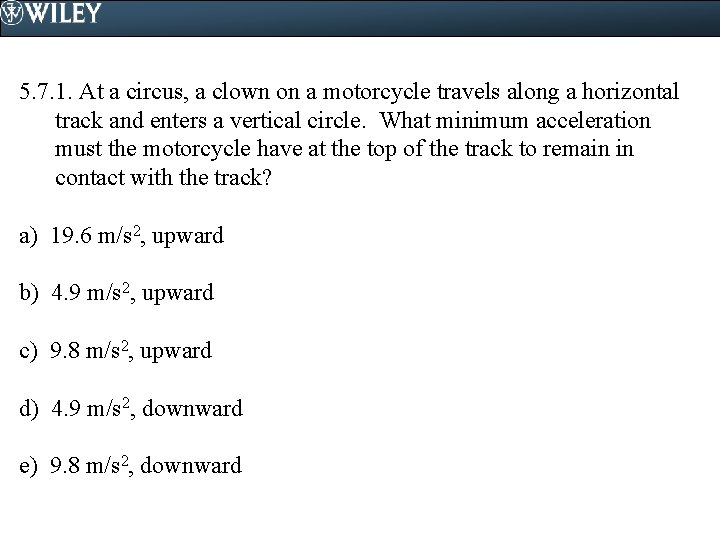5. 7. 1. At a circus, a clown on a motorcycle travels along a horizontal track and enters a vertical circle. What minimum acceleration must the motorcycle have at the top of the track to remain in contact with the track? a) 19. 6 m/s 2, upward b) 4. 9 m/s 2, upward c) 9. 8 m/s 2, upward d) 4. 9 m/s 2, downward e) 9. 8 m/s 2, downward5. 7. 1. At a circus, a clown on a motorcycle travels along a horizontal track and enters a vertical circle. What minimum acceleration must the motorcycle have at the top of the track to remain in contact with the track? a) 19. 6 m/s 2, upward b) 4. 9 m/s 2, upward c) 9. 8 m/s 2, upward d) 4. 9 m/s 2, downward e) 9. 8 m/s 2, downward